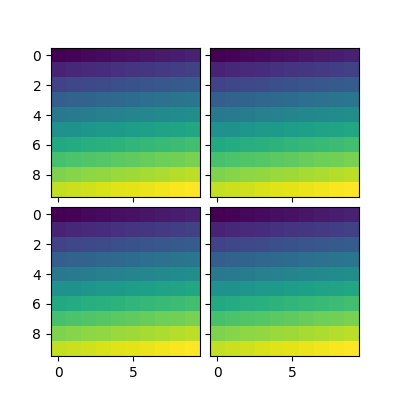Travis-CI:# Simple Axesgrid¶```import matplotlib.pyplot as plt
from mpl_toolkits.axes_grid1 import ImageGrid
import numpy as np

im = np.arange(100).reshape((10, 10))

fig = plt.figure(1, (4., 4.))
grid = ImageGrid(fig, 111,  # similar to subplot(111)
nrows_ncols=(2, 2),  # creates 2x2 grid of axes